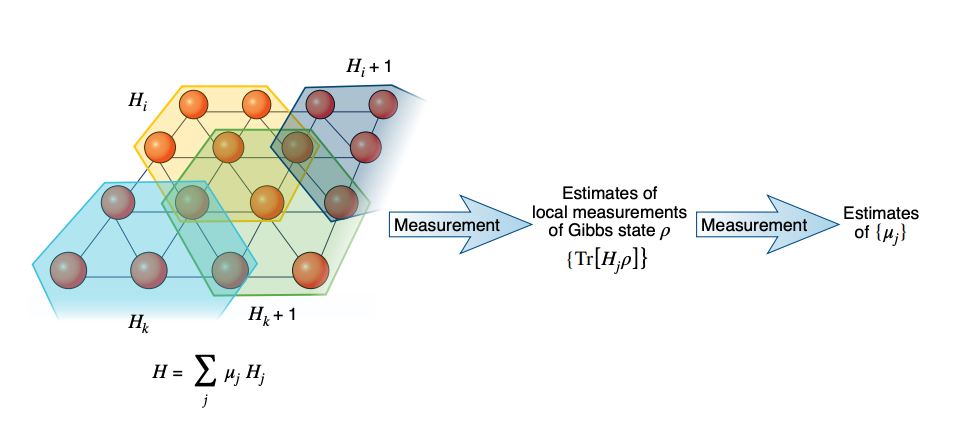02 Jun 2021
Research
6 minute read

# An efficient method to learn quantum many-body systems

Through a mathematical tour-de-force, the IBM Quantum's team has proven the existence of a machine learning algorithm that can describe quantum systems with many particles using fewer measurements than any previous algorithm. This algorithm has the potential to play a role in the verification of upcoming quantum technologies and probing quantum matter.

Systems of particles are generally very complex to understand at the most detailed level of their quantum behavior. At thermal equilibrium, where net heat doesn't flow into or out of the system, physicists describe quantum systems using Gibbs states, which contain information about all the interactions between the quantum particles. In this paper, the researchers ask, if given access to repeated measurements of the Gibbs states, whether one can learn the underlying interactions in those systems, in the form of quantum physics' central mathematical operator to describe the system, called the Hamiltonian.Figure 1:Geometrically local Hamiltonians defined by weighted local terms. The Hamiltonian, H, of a physical system (red circles) on a lattice can be written as a sum of local terms Hj that act on a localized spatial region (blue, yellow, green and grey cells). The contribution of each local term is determined by a weight coefficient μj. Measuring the thermal Gibbs state of the system using each of the local terms, which all correspond to observable quantities, provides enough information for the weights μj to be determined accurately, and thus to reconstruct the Hamiltonian. (Credit: Dunjko, V. Inside quantum black boxes. Nat. Phys. (2021).)

Scientists have already proven efficient ways to tackle the classical version of this problem, where the information about the system arrives as strings of zeros and ones. However, using methods to calculate the richer quantum version—requiring the algorithm to learn quantum interactions—has remained out of reach for quantum algorithm developers and has been a long-standing open question. That is, until now.

Plenty of proposals exist to use machine learning algorithms to learn quantum interactions—however, none of these methods have achieved what can be done in the classical world. This paper1 is the first to prove that machine learning techniques can learn quantum interactions—and the first to provably do so more efficiently than any earlier technique. Prior to this work, the best algorithms for solving the Hamiltonian problem took exponentially more measurements as the problem size increased, but this new algorithm only requires a cubic increase in the number of measurements. More simply, given a system of n particles, previous techniques would require in the range of 2n measurements, while the new algorithm would require only about n3. This difference quickly becomes significant as the problem grows larger.

The major roadblock to achieving this result are the bizarre quantum effects in many-body systems. In the classical world and at some fixed temperature, the state of a particle is independent of all other particles even when we know the state of its neighbors, thanks to a property known as the “Markov condition.” This is the very feature that allows machine learning techniques to be successfully applied to infer classical systems such as the Ising model (which describes magnetic systems as a collection of two-state objects). Surprisingly, the quantum world violates this property, and the previous attempts to develop the learning algorithm did not know how to proceed without it.

However, the team found a workaround such that they did not have to prove the quantum Markov property in order to prove their algorithm's ability. Instead, they found that they needed only to prove that the partition function of the Gibbs state, which defines the system's statistical properties, satisfied strong convexity—small changes in the parameters of the interactions lead to notable changes in the overall state.

The team proved this successfully, demonstrating that the simplest quantum measurements could characterize these Gibbs states. The article describes this work was recently published in Nature Physics by Srinivasan Arunachalam, alongside coauthors Anurag Anshu from the University of California, Berkeley, Mehdi Soleimanifar from the Massachusetts Institute of Technology and Tomotaka Kuwahara from the RIKEN Center for Advanced Intelligence Project (AIP).

Finding a system's Hamiltonian based on its Gibb's state is an important problem in quantum computing with plenty of useful applications. First and foremost, it's useful to verify that a quantum computer relying on preparing and manipulating Gibbs states is working the way that you hoped. But in the future, this algorithm will allow scientists to use quantum measurements to explore the properties of different quantum materials. Perhaps soon, Qiskit users will implement this algorithm on near-term devices.

Ultimately, these kinds of calculations are crucial to pushing the field forward. The more quantum learning methods we find, the more convincing quantum computing becomes as a useful tool to probe nature. Now, thanks to this algorithm, we're even more confident of the potential of machine learning techniques and quantum computing to study complex quantum systems.

02 Jun 2021